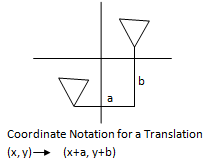Name: ___________________Date:___________________

 Email us to get an instant 20% discount on highly effective K-12 Math & English kwizNET Programs!

### Geometry7.4 Translation

 Translation Mapping: A mapping of a plane in which each point of the plane moves in the same direction by the segments, all congruent to a given segment (equal distance) is called a translation. Translation moves every point of a figure the same distance in the same direction.Examples: Find the coordinates of the image of (2,7), under the translation defined by (x,y) ® (x+2, y+1). T(x,y) ® (x+2, y+1). T(2,7) ® (2+2, 7+1). The coordinates of the image is (4,8) A quadrilateral has vertices A(-4,3), B(-2,4), C(-1,1) and D(-3,1). Find the coordinates of the image after translation (x,y) ® (x+5,y-2). (x,y) ® (x+2, y+1). A(-4,3) ® (1, 1) B(-2,4) ® (3, 2) C(-1,1) ® (4, -1) D(-3,1) ® (2, -1) Directions: Choose the correct answer. Also write at least 5 examples of your own.Name: ___________________Date:___________________

### Geometry7.4 Translation

 Q 1: Find the coordinates of the image of (2, 8), under the translation defined by (x,y) ® (x, y-5).(3, 4)(4, 3)(3, -4) Q 2: Point on image: (4, 0); translation: (x,y) --> (x+2, y-3) Find the corresponding point on the original figure. (3, 4)(2, 3)(4, 3) Q 3: Find the coordinates of the image of (4, 9), under the translation defined by (x,y) ® (x+4, y-2).(6, 8)(8, 7)(7, 8) Q 4: Point on image: (6, -9); translation: (x,y) --> (x-7, y-4) Find the corresponding point on the original figure. (13, -5)(-4, 10)(-1,-13) Q 5: Find the coordinates of the image of (5, 3), under the translation defined by (x,y) ® (x-2, y-4).(-3, 1)(3, -1)(3, 1) Q 6: If (0,3) translates to (1,2), then (2,5) translates to _______(3, 4)(4, 3)(2, 5) Question 7: This question is available to subscribers only! Question 8: This question is available to subscribers only!

#### Subscription to kwizNET Learning System costs less than \$1 per month & offers the following benefits:

• Unrestricted access to grade appropriate lessons, quizzes, & printable worksheets
• Instant scoring of online quizzes
• Progress tracking and award certificates to keep your student motivated
• Unlimited practice with auto-generated 'WIZ MATH' quizzes